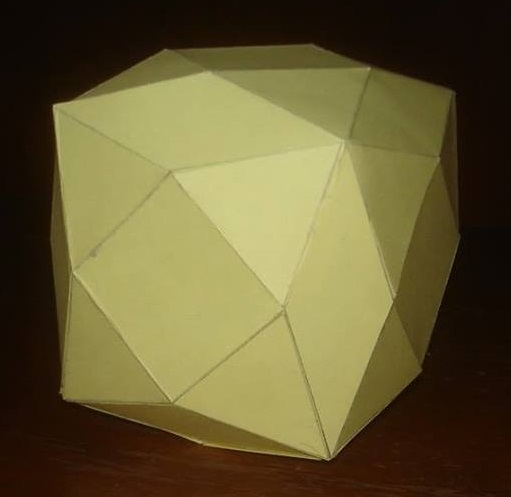Acronym ... Name rhombical octa-augmented truncated-octehedral variant `©` Vertex figure [3,4,3,R], [(3,r)3] Coordinates (sqrt(8)/3, sqrt(8)/3, sqrt(8)/3)     all changes of sign (vertex inscribed cube) (5 sqrt(2)/6, 1/sqrt(2), 0)             all permutations, all changes of sign (vertex inscribed truncated-octehedral variant) Confer expanded 8-augmented toe variant

The short diagonal RR of the rhombs ((r,R)2) is 2/3 edge units, the long one rr then 4 sqrt(2)/3 edge units.
Their acute angle is r = arccos(7/9) = 38.942441°, the obtuse angle thus is R = arccos(-7/9) = 141.057559°.

Incidence matrix

```24 * |  2  2 | 1  2  1  [3,4,3,R]
* 8 |  0  6 | 0  3  3  [(3,r)3]
-----+-------+--------
2 0 | 24  * | 1  1  0
1 1 |  * 48 | 0  1  1
-----+-------+--------
4 0 |  4  0 | 6  *  *  {4}
2 1 |  1  2 | * 24  *  {3}
2 2 |  0  4 | *  * 12  ((r,R)2)
```